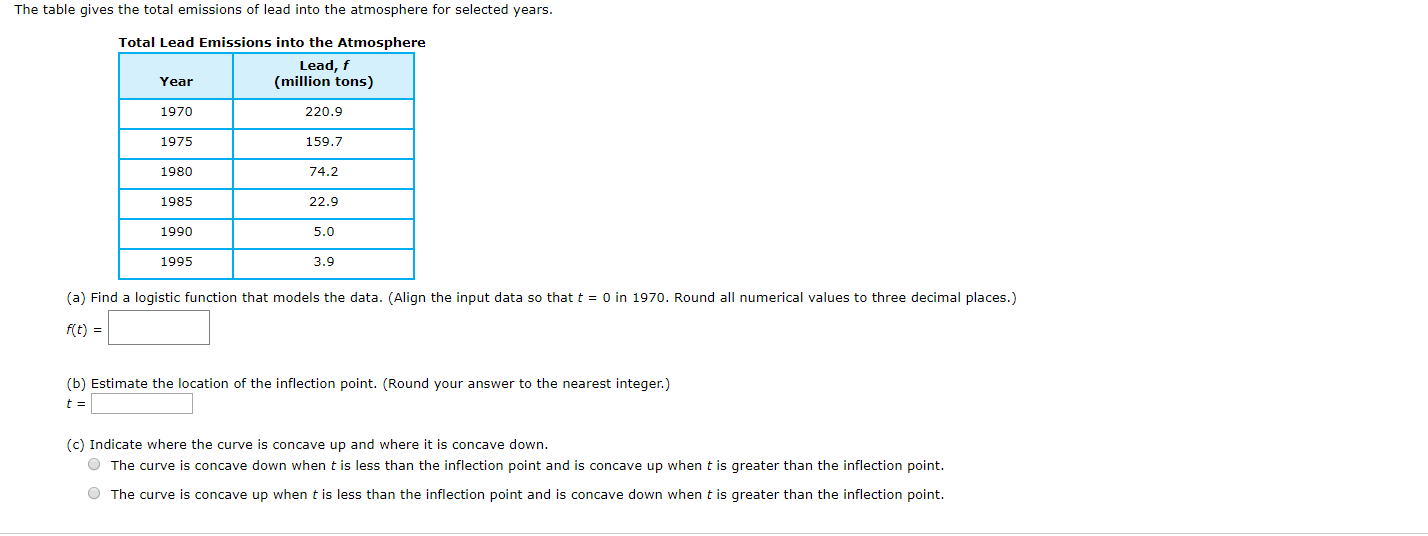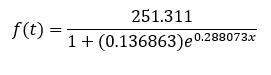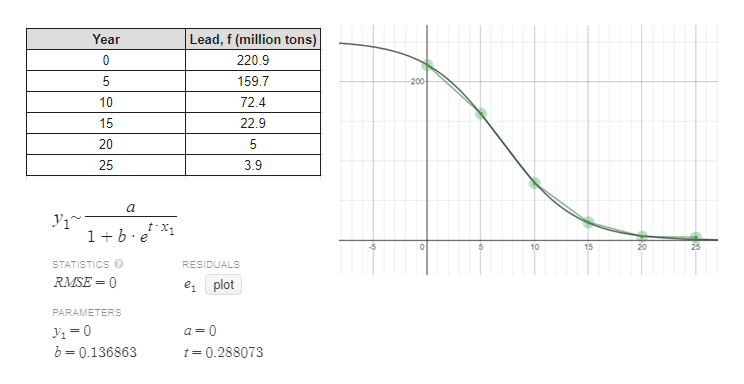# The table gives the total emissions of lead into the atmosphere for selected years.Total Lead Emissions into the AtmosphereLead, f(million tons)Year1970220.9159.71975198074.222.9198519905.019953.9(a) Find a logistic function that models the data. (Align the input data so that t 0 in 1970. Round all numerical values to three decimal places.)f(t)(b) Estimate the location of the inflection point. (Round your answer to the nearest integer.)t =(c) Indicate where the curve is concave up and where it is concave down.OThe curve is concave down when t is less than the inflection point and is concave up when t is greater than the inflection point.OThe curve is concave up when t is less than the inflection point and is concave down when t is greater than the inflection point.

Question
250 viewshelp_outlineImage TranscriptioncloseThe table gives the total emissions of lead into the atmosphere for selected years. Total Lead Emissions into the Atmosphere Lead, f (million tons) Year 1970 220.9 159.7 1975 1980 74.2 22.9 1985 1990 5.0 1995 3.9 (a) Find a logistic function that models the data. (Align the input data so that t 0 in 1970. Round all numerical values to three decimal places.) f(t) (b) Estimate the location of the inflection point. (Round your answer to the nearest integer.) t = (c) Indicate where the curve is concave up and where it is concave down. OThe curve is concave down when t is less than the inflection point and is concave up when t is greater than the inflection point. OThe curve is concave up when t is less than the inflection point and is concave down when t is greater than the inflection point. fullscreen
check_circle

Step 1

(a) Let us align the input data so that t = 0 in 1970.

Step 2

The logistic model is shown below:help_outlineImage TranscriptioncloseLead, f (million tons) Year 0 220.9 159.7 5 -200 72.4 10 15 22.9 20 5 25 3.9 a 1 b x 10 20 15 STATISTICS RESIDUALS RMSE-0 e plot PARAMETERS 0 b 0.136863 a 0 t 0.288073 fullscreen
Step 3

(b) Inflation point is where the curvature of the graph changes direction. Here, we can see that at t = 8 the graph changes dire...

### Want to see the full answer?

See Solution

#### Want to see this answer and more?

Solutions are written by subject experts who are available 24/7. Questions are typically answered within 1 hour.*

See Solution
*Response times may vary by subject and question.
Tagged in

### Other